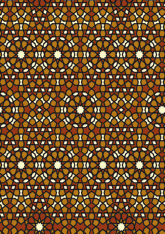# Selimiye Mosque, Edirnedata193/TUR0108

## Geometry

• Growth dynamic build of this pattern.
• Random dynamic build of this pattern.
• Structured dynamic build of this pattern.
• The symmetry group of the tiling is 2*22 (cmm).
• All the internal angles of the constituent polygons are a multiple of 36°.
• Contains 31 regular two-pointed star polygons with vertex angle of 72°.
• Contains 40 regular pentagons.
• Contains five regular five-pointed star polygons with vertex angle of 72°.
• Contains four regular 10-pointed star polygons with vertex angle of 72°.
• There are 14 non-regular reflective tiles (including one kite).
• The tiling satisfies the two-colour condition with straight cross-overs, but interlace counts not determined.
• The tiling is edge-to-edge.
• As drawn, contains about 2063 polygons.

## References

Publications referenced: